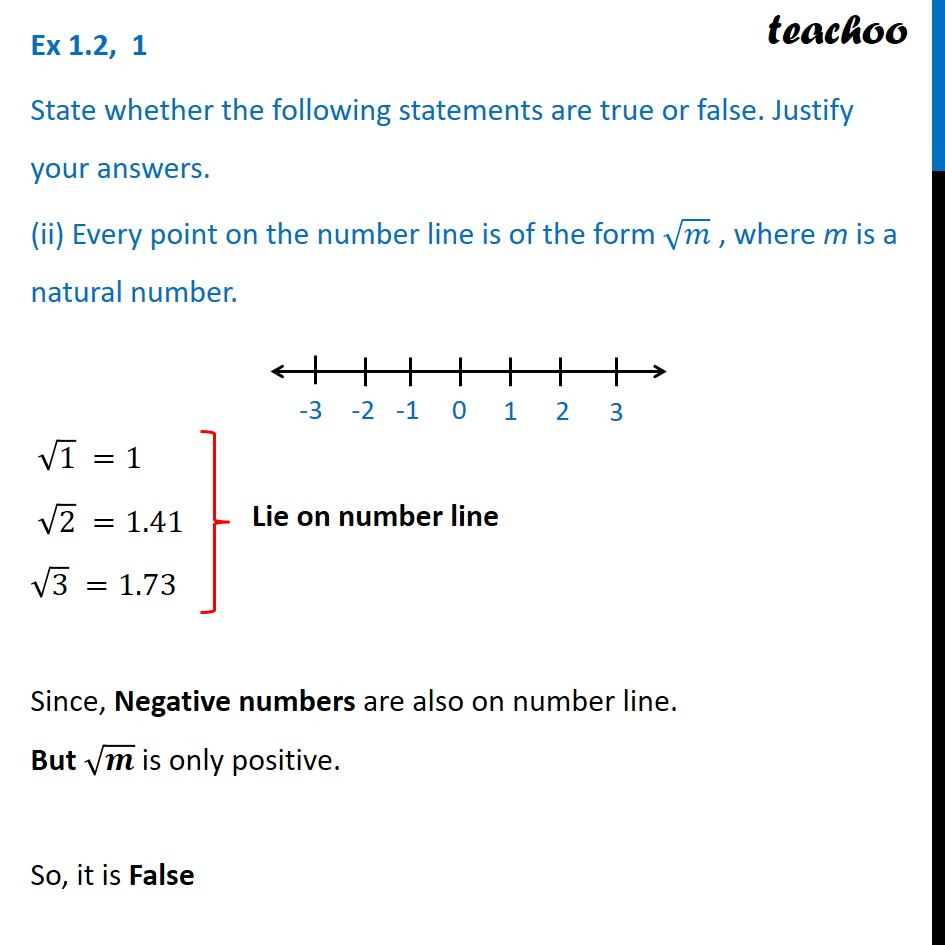Ex 1.2

Chapter 1 Class 9 Number Systems
Serial order wiseLearn in your speed, with individual attention - Teachoo Maths 1-on-1 Class

### Transcript

Ex 1.2, 1 State whether the following statements are true or false. Justify your answers. (ii) Every point on the number line is of the form √𝑚 , where m is a natural number. √1 =1 √2 =1.41 √3 =1.73 Since, Negative numbers are also on number line. But √𝒎 is only positive. So, it is False Lie on number line